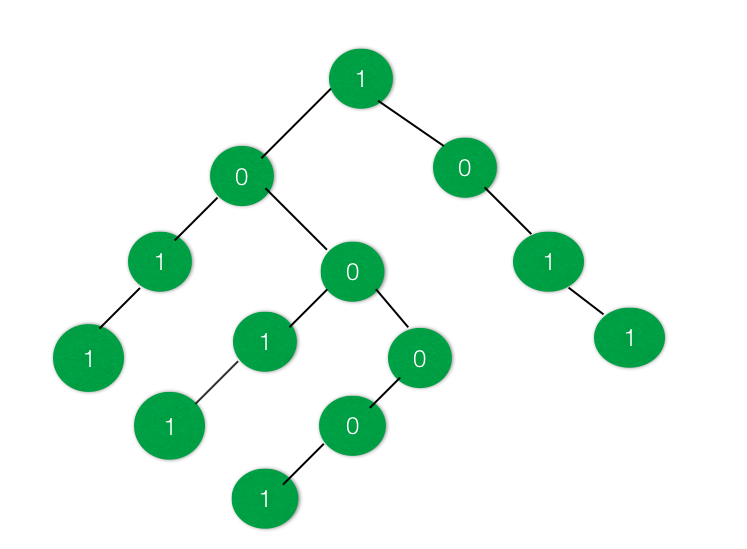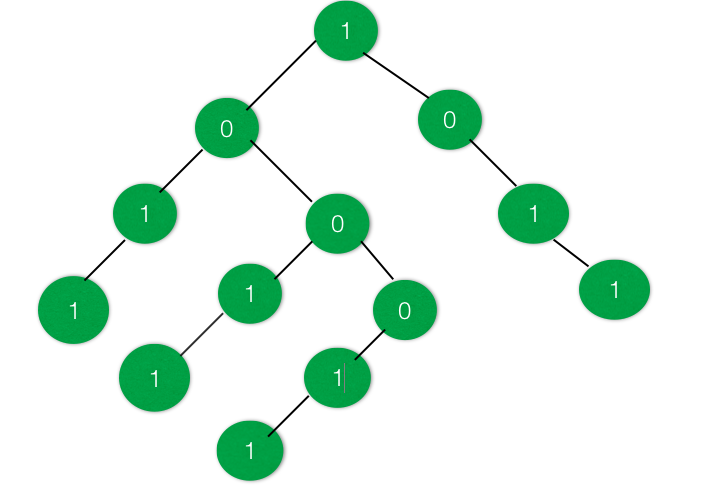# Check if the given Binary Tree have a Subtree with equal no of 1’s and 0’s

Given a Binary Tree having data at nodes as either 0’s or 1’s. The task is to find out whether there exists a subtree having an equal number of 1’s and 0’s.

Examples:

Input :Output : True
There are two subtrees present in the above tree where the number of 1’s is equal to the number of 0’s.

Input :Output : False
There is no such subtree present which has the number of 1’s equal to number of 0’s

Approach: The idea is to change the data 0’s of the tree to -1. So that it becomes very easy to find the subtree having equal number of 0’s and 1’s. After converting all 0’s to -1, create a sum tree. After creating the sum tree, each node will contain the sum of all node lying under it.

Traverse the tree again and find if there is a node having 0 sum, it means that there is a subtree that has the equal number of 1’s and -1’s, i.e. equal number of 1’s and 0’s.

Below is the implementation of the above approach:

 `// C++ program to check if there exist a ` `// subtree with equal number of 1's and 0's ` ` `  `#include ` `using` `namespace` `std; ` ` `  `// Binary Tree Node ` `struct` `node { ` `    ``int` `data; ` `    ``struct` `node *right, *left; ` `}; ` ` `  `// Utility function to create a new node ` `struct` `node* newnode(``int` `key) ` `{ ` `    ``struct` `node* temp = ``new` `node; ` `    ``temp->data = key; ` `    ``temp->right = NULL; ` `    ``temp->left = NULL; ` ` `  `    ``return` `temp; ` `} ` ` `  `// Function to convert all 0's in the ` `// tree to -1 ` `void` `convert(``struct` `node* root) ` `{ ` `    ``if` `(root == NULL) { ` `        ``return``; ` `    ``} ` ` `  `    ``// Move to right subtree ` `    ``convert(root->right); ` ` `  `    ``// Replace the 0's with -1 in the tree ` `    ``if` `(root->data == 0) { ` `        ``root->data = -1; ` `    ``} ` ` `  `    ``// Move to left subtree ` `    ``convert(root->left); ` `} ` ` `  `// Function to convert the tree to a SUM tree ` `int` `sum_tree(``struct` `node* root) ` `{ ` `    ``int` `a = 0, b = 0; ` ` `  `    ``if` `(root == NULL) { ` `        ``return` `0; ` `    ``} ` ` `  `    ``a = sum_tree(root->left); ` `    ``b = sum_tree(root->right); ` ` `  `    ``root->data = root->data + a + b; ` ` `  `    ``return` `root->data; ` `} ` ` `  `// Function to check if there exists a subtree ` `// with equal no of 1s and 0s ` `int` `checkSubtree(``struct` `node* root, ``int` `d) ` `{ ` `    ``if` `(root == NULL) { ` `        ``return` `0; ` `    ``} ` ` `  `    ``// Check if there is a subtree with equal ` `    ``// 1s and 0s or not ` `    ``if` `(d == 0) { ` `        ``d = checkSubtree(root->left, d); ` `    ``} ` ` `  `    ``if` `(root->data == 0) { ` `        ``d = 1; ` `        ``return` `d; ` `    ``} ` ` `  `    ``if` `(d == 0) { ` `        ``d = checkSubtree(root->right, d); ` `    ``} ` ` `  `    ``return` `d; ` `} ` ` `  `// Driver Code ` `int` `main() ` `{ ` `    ``// Create the Binary Tree ` `    ``struct` `node* root = newnode(1); ` `    ``root->right = newnode(0); ` `    ``root->right->right = newnode(1); ` `    ``root->right->right->right = newnode(1); ` `    ``root->left = newnode(0); ` `    ``root->left->left = newnode(1); ` `    ``root->left->left->left = newnode(1); ` `    ``root->left->right = newnode(0); ` `    ``root->left->right->left = newnode(1); ` `    ``root->left->right->left->left = newnode(1); ` `    ``root->left->right->right = newnode(0); ` `    ``root->left->right->right->left = newnode(0); ` `    ``root->left->right->right->left->left = newnode(1); ` ` `  `    ``// Convert all 0s in tree to -1 ` `    ``convert(root); ` ` `  `    ``// Convert the tree into a SUM tree ` `    ``sum_tree(root); ` ` `  `    ``// Check if required Subtree exists ` `    ``int` `d = 0; ` `    ``if` `(checkSubtree(root, d)) { ` `        ``cout << ``"True"` `<< endl; ` `    ``} ` `    ``else` `{ ` `        ``cout << ``"False"` `<< endl; ` `    ``} ` ` `  `    ``return` `0; ` `} `

Output:

```True
```

Time Complexity: O(N)
Space Complexity: O(1)

My Personal Notes arrow_drop_upCheck out this Author's contributed articles.

If you like GeeksforGeeks and would like to contribute, you can also write an article using contribute.geeksforgeeks.org or mail your article to contribute@geeksforgeeks.org. See your article appearing on the GeeksforGeeks main page and help other Geeks.

Please Improve this article if you find anything incorrect by clicking on the "Improve Article" button below.

Article Tags :
Practice Tags :

Be the First to upvote.

Please write to us at contribute@geeksforgeeks.org to report any issue with the above content.